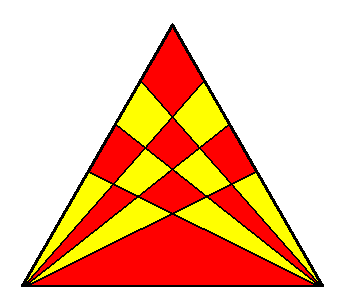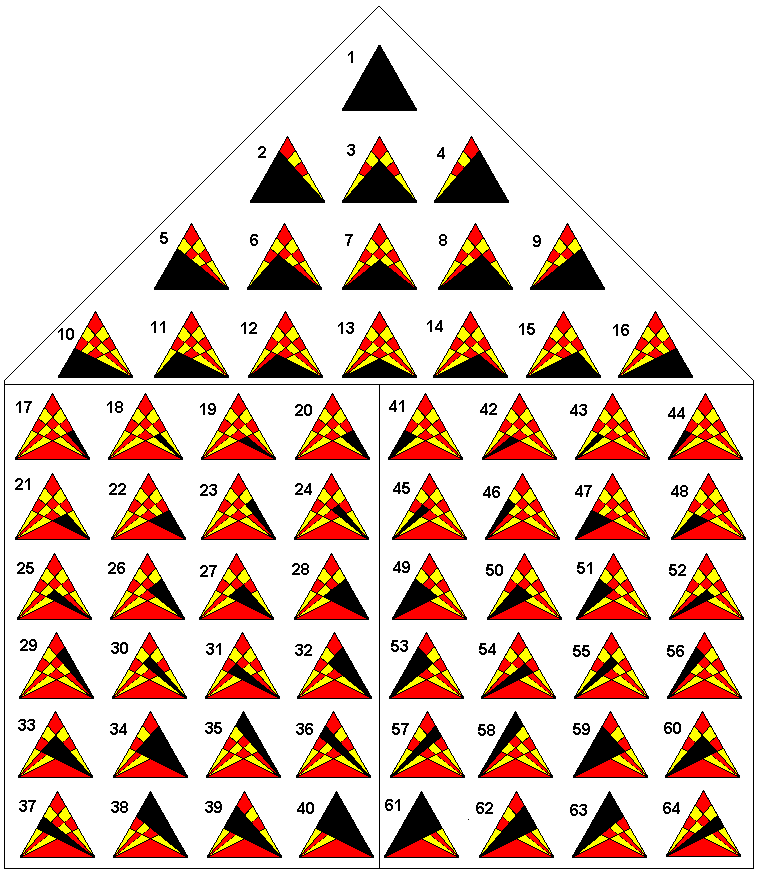## HOW MANY TRIANGLES?How many triangles can you count in this diagram?

I got this puzzle from a book of puzzles. I don't often do that but my reason for presenting it to you this month is that I think the answer in the back of the book is wrong. The answer given is 64 but my total is less than this. Can you count as many as 64? Let me know.

The prize will go to the person who gets the right answer, which is not necessarily my answer but I have to be convinced it is right. It could be 64, you never know!

David Broughton.

Scroll down to see the answer.

 ANSWER: The right answer is, in fact, 64 -- the answer in the book was RIGHT!!!Triangles 1 to 16 all include the base of the equilateral triangle. In counting the triangles, separating this set out from the others avoids confusions over which to count twice due to symmetry. Triangles 17 to 40 are 24 triangles that include the right hand corner and do not include the base triangles of the first 16. Triangles 41 to 64 are a mirror image of triangles 17 to 40. The general formula is very simple. It is (n+1)3 where n is the number of lines from each base corner to the opposite side inside the equilateral triangle (in our case, n=3). So for n=0 there is one triangle; 8 triangles for n=1 and so on. The n=1 case is easy to check by counting without making a mistake. My original answer was 40 and then I rethought it, again incorrectly, getting 76. It was in trying to work out the general formula that things started to fall into place and I was able to separate out the set of 16 base triangles from those that could be doubled due to symmetry. The 16 base triangles have a symmetry as well of a different kind (see diagram) which helps to confuse the issue but I think the diagram settles the issue conclusively. ANSWERS FROM MEMBERS This puzzle was unusually popular. The answers were also unusually varied, ranging from 34 (the lowest) to 80 (the highest). I had 8 entries, one entrant supplying two rethinks, but all three tries were wrong! The three who gave the answer 64 were John Stafford, John Bownas and Dennis Linzmaier with John Stafford winning the random draw. Thank you to all the others who took part to make this a very interesting puzzle. And thank you also for the analyses and diagrams I received that confirmed the correct answer.

[home] [back]